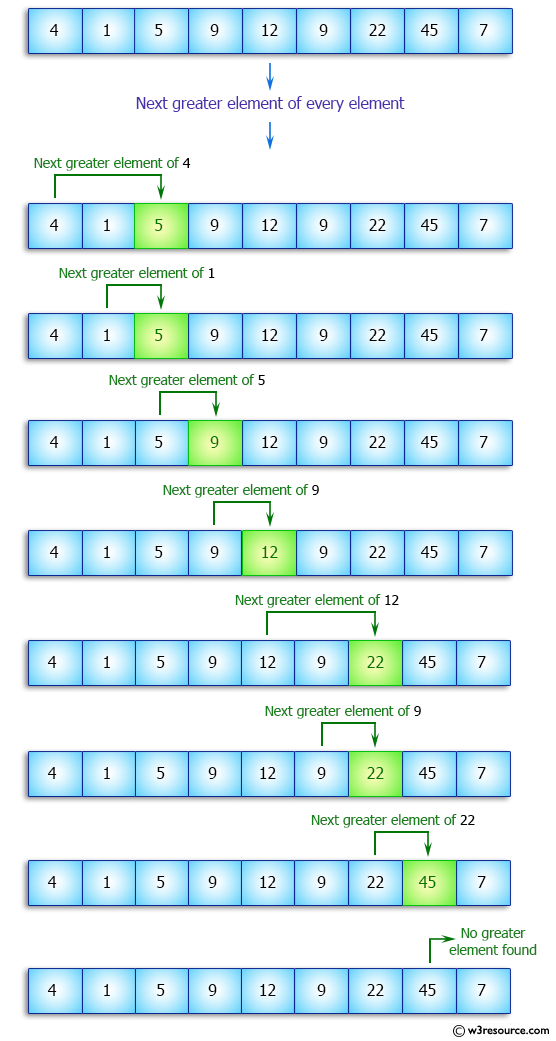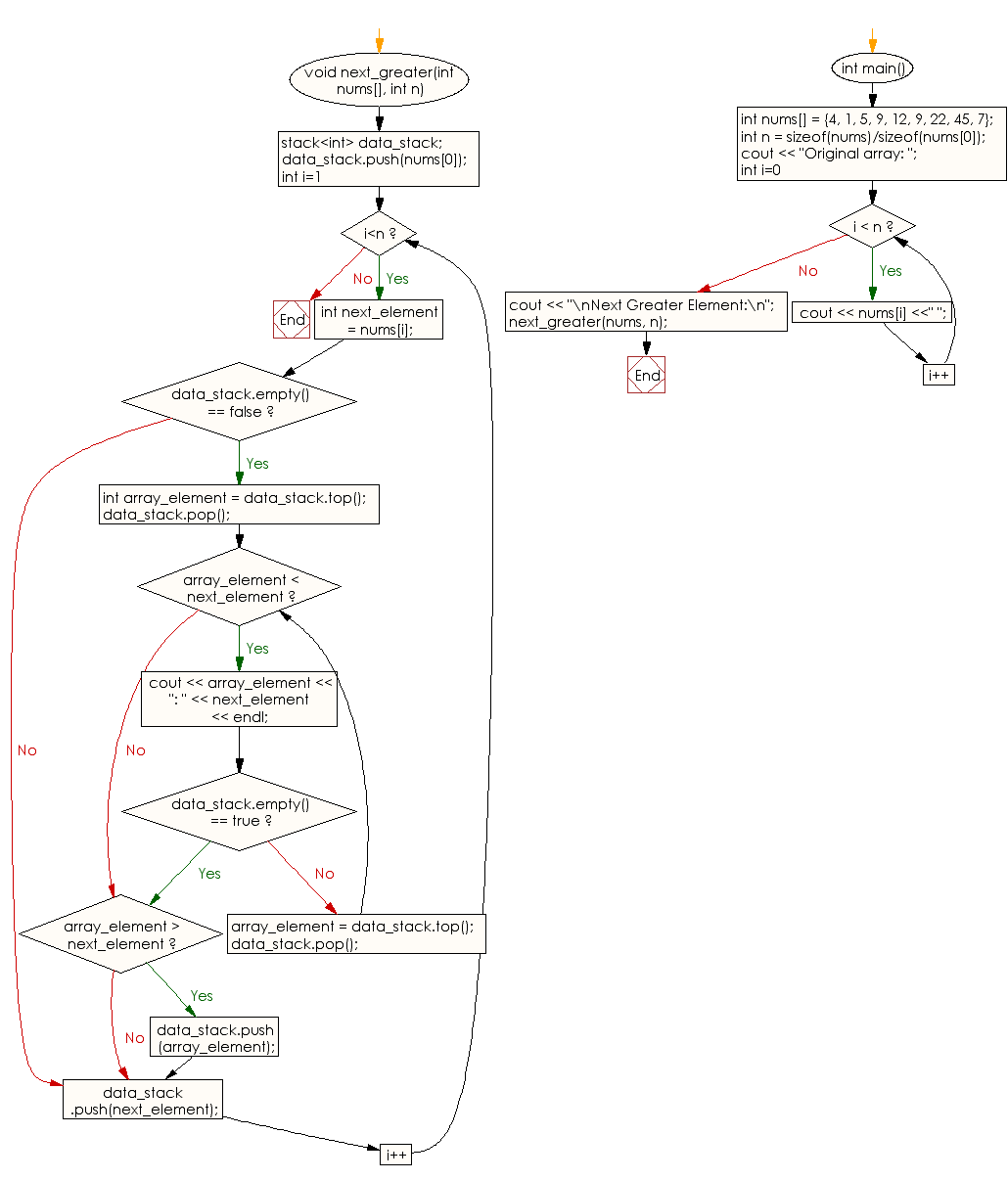﻿ C++ : Next greater element of every element of an array# C++ Exercises: Find the next greater element of every element of a given array of integers

## C++ Array: Exercise-8 with Solution

Write a C++ program to find the next greater element of every element of a given array of integers. Ignore those elements which have no greater element.

Pictorial Presentation:Sample Solution:

C++ Code :

``````#include<iostream>
using namespace std;

void next_greater(int nums[], int n)
{
stack<int> data_stack;

data_stack.push(nums);

for (int i=1; i<n; i++)
{
int next_element = nums[i];

if (data_stack.empty() == false)
{
int array_element = data_stack.top();
data_stack.pop();

while (array_element < next_element)
{
cout << array_element << ": " << next_element
<< endl;
if (data_stack.empty() == true)
break;
array_element = data_stack.top();
data_stack.pop();
}

if (array_element > next_element)
data_stack.push(array_element);
}

data_stack.push(next_element);
}

}
int main()
{
int nums[] = {4, 1, 5, 9, 12, 9, 22, 45, 7};
int n = sizeof(nums)/sizeof(nums);
cout << "Original array: ";
for (int i=0; i < n; i++)
cout << nums[i] <<" ";
cout << "\nNext Greater Element:\n";
next_greater(nums, n);
}
``````

Sample Output:

```Original array: 4 1 5 9 12 9 22 45 7
Next Greater Element:
1: 5
4: 5
5: 9
9: 12
9: 22
12: 22
22: 45
```

Flowchart:C++ Code Editor:

What is the difficulty level of this exercise?

Test your Programming skills with w3resource's quiz.

﻿